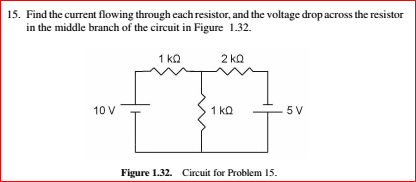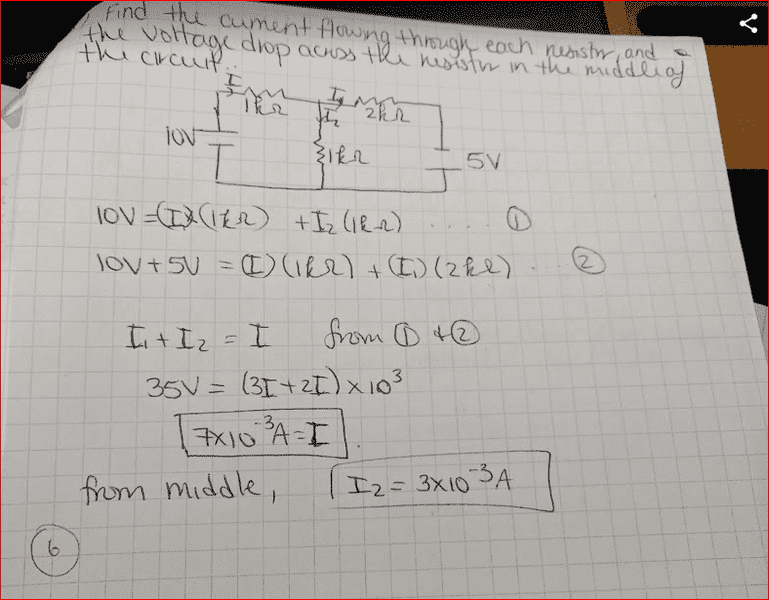# Find the Current Flowing through each resistor

## Homework Statement## Homework Equations

/ 3. The Attempt at a Solution
Looking to solve mistakes to study from homework. Here's what I had so far[/B]BvU
Homework Helper
Good. Now: what is your question ?

The batteries are arranged in opposite polarity. Check your second equation.

I'm just wondering how to find the Voltage drop.
Would I need to find the voltage using V=IR for each of the currents that I found and then I could observe the drop from V1 to V2?

BvU
Homework Helper
IS it clear what you mean with V1 and V2 ? What do you think of Magoo's remark ?

The batteries are arranged in opposite polarity. Check your second equation.
Aren't the currents going in the same direction, though? - They're both moving in a clock-wise direction?

BvU
Homework Helper
Yes, so do the voltage drop over the 2 k##\Omega## and the 5V have he same sign or an opposite sign ?

Hmm...If you start with 10V and on the opposite side is 5V, is it bc of Kirchhoff's voltage law that the voltage drop would be negative over the 2kOhm to 5V battery?
Because your overall voltage of the loop should end up =0?

cnh1995
Homework Helper
Gold Member
Because your overall voltage of the loop should end up =0?
Sum of potential changes (gains and drops) should be zero.

If you start with 10V and on the opposite side is 5V, is it bc of Kirchhoff's voltage law that the voltage drop would be negative over the 2kOhm to 5V battery
Usually, you can't predict that. You should assume the directions of currents first and solve the KVL and KCL equations. If the current direction assumed is incorrect, you'll get a minus sign in the answer.

A few things:

Voltage polarity is due to potential difference.

Your equations don't seem to be loop (mesh) equations.

The direction to assign a current for analysis is arbitrary, as long as you don't swap it's direction mid analysis. If you guessed the correct direction, the result will be positive. Otherwise, the result will be negative. Compare your result, to the direction you assigned, and that will tell you which way current is flowing.